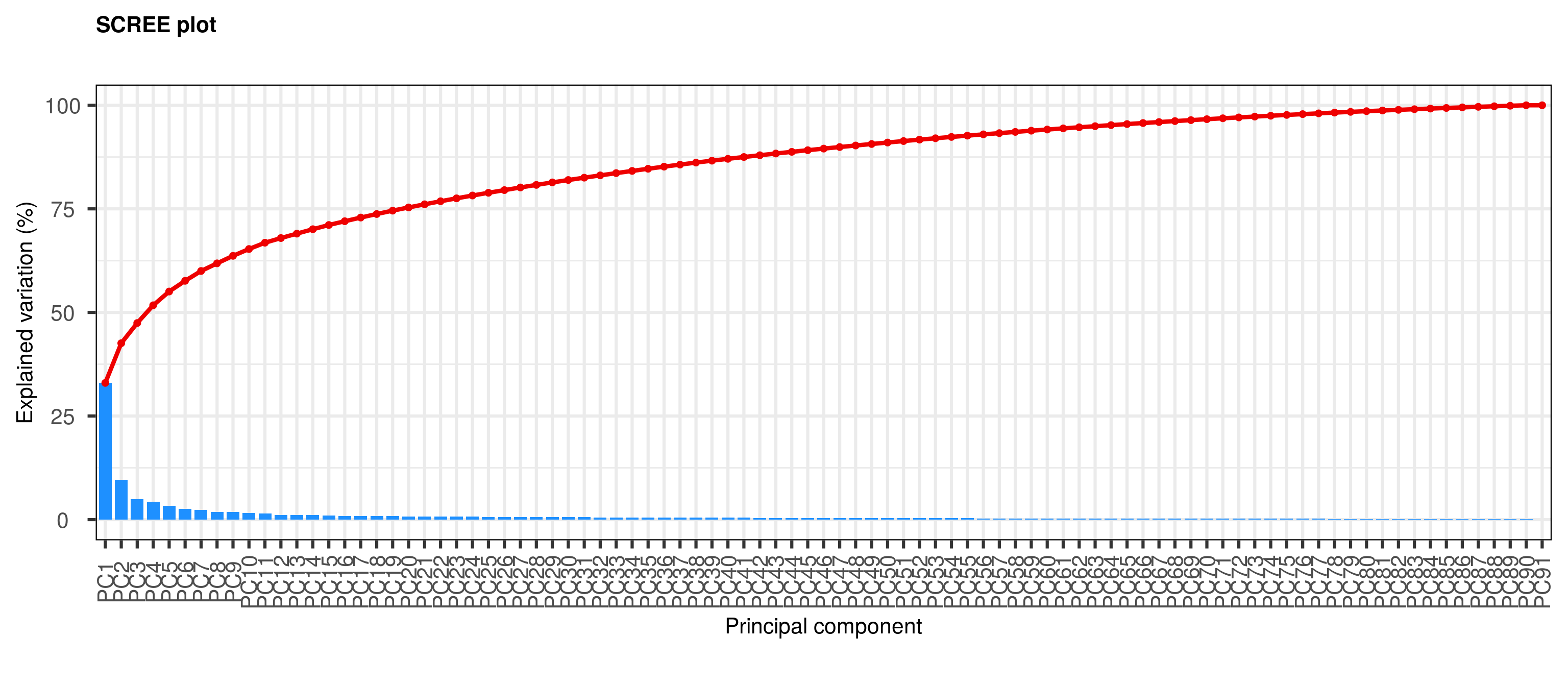# 1 Introduction

Principal Components Analysis (PCA) is a very powerful technique that has wide applicability in data science, bioinformatics, and further afield. It was initially developed to analyse large volumes of data in order to tease out the differences/relationships between the logical entities being analysed. It extracts the fundamental structure of the data without the need to build any model to represent it. This ‘summary’ of the data is arrived at through a process of reduction that can transform the large number of variables into a lesser number that are uncorrelated (i.e. the ‘principal components’), whilst at the same time being capable of easy interpretation on the original data (Blighe 2013) (Blighe and Lewis 2018).

# 2 Installation

``````    if (!requireNamespace('BiocManager', quietly = TRUE))
install.packages('BiocManager')
BiocManager::install('PCAtools')``````

Note: to install development version:

``    devtools::install_github('kevinblighe/PCAtools')``

## 2.2 2. Load the package into R session

``    library(PCAtools)``

# 3 Quick start

For this vignette, we will load breast cancer gene expression data with recurrence free survival (RFS) from Gene Expression Profiling in Breast Cancer: Understanding the Molecular Basis of Histologic Grade To Improve Prognosis.

First, let’s read in and prepare the data:

``````  library(Biobase)
library(GEOquery)

# load series and platform data from GEO
gset <- getGEO('GSE2990', GSEMatrix =TRUE, getGPL=FALSE)

x <- exprs(gset[])

# remove Affymetrix control probes
x <- x[-grep('^AFFX', rownames(x)),]

# extract information of interest from the phenotype data (pdata)
idx <- which(colnames(pData(gset[])) %in%
c('age:ch1', 'distant rfs:ch1', 'er:ch1',
'time rfs:ch1'))

row.names = rownames(pData(gset[])))

# tidy column names
'Size', 'Time.RFS')

# prepare certain phenotypes

# remove samples from the pdata that have any NA value

# filter the expression data to match the samples in our pdata

# check that sample names match exactly between pdata and expression data
``##  TRUE``

Conduct principal components analysis (PCA)

``  p <- pca(x, metadata = metadata, removeVar = 0.1)``

## 3.1 A SCREE plot

``  screeplot(p)``Figure 1: A SCREE plot to show the proportion of explained variance by PC

## 3.2 A bi-plot

``  biplot(p)``